Xie, W., Wang, Z., Zhang, Z., and Wang, Z. (2021). "Dynamic test of laminated veneer lumber elastic modulus and its probability distribution," BioResources 16(2), 3318-3327.

#### Abstract

The vibrational frequency method was used to measure the elastic modulus of laminated veneer lumber (LVL), and the feasibility of using Weibull distribution to analyze the elastic modulus data of LVL was considered. Samples were randomly selected as test pieces at the factory. The sponge support structure was used to realize the free beam state, and the modal test results verified the accuracy of realizing the free beam. Under transient excitation, the elastic modulus of the specimen was obtained by testing the first-order bending frequency. The Weibull distribution fitting test, Weibull distribution K-S test, and normal distribution K-S test were used for the test data. The probability of LVL elastic modulus was calculated under a given value. The results showed that the LVL elastic modulus did not obey the two-parameter Weibull distribution (Eu=0). The LVL elastic modulus fit to the three-parameter Weibull distribution (Eu) was greater than half of the minimum test value and the normal distribution. When 9 GPa and 8 GPa were used as the setting values of Eu, the calculated probability value was relatively stable. At this time, Eu was 81% and 92% of the minimum elastic modulus 9.815 GPa. Therefore, it was recommended to use 80% to 90% of the minimum value of the measured data as the setting value of the position parameter Eu. The three-parameter Weibull distribution and the normal distribution calculated LVL elastic modulus have the same probability under the given value.

Dynamic Test of Laminated Veneer Lumber Elastic Modulus and Its Probability Distribution

Wenbo Xie, Zi Wang, Zhiqiang Zhang,* and Zheng Wang

The vibrational frequency method was used to measure the elastic modulus of laminated veneer lumber (LVL), and the feasibility of using Weibull distribution to analyze the elastic modulus data of LVL was considered. Samples were randomly selected as test pieces at the factory. The sponge support structure was used to realize the free beam state, and the modal test results verified the accuracy of realizing the free beam. Under transient excitation, the elastic modulus of the specimen was obtained by testing the first-order bending frequency. The Weibull distribution fitting test, Weibull distribution K-S test, and normal distribution K-S test were used for the test data. The probability of LVL elastic modulus was calculated under a given value. The results showed that the LVL elastic modulus did not obey the two-parameter Weibull distribution (Eu=0). The LVL elastic modulus fit to the three-parameter Weibull distribution (Eu) was greater than half of the minimum test value and the normal distribution. When 9 GPa and 8 GPa were used as the setting values of Eu, the calculated probability value was relatively stable. At this time, Eu was 81% and 92% of the minimum elastic modulus 9.815 GPa. Therefore, it was recommended to use 80% to 90% of the minimum value of the measured data as the setting value of the position parameter Eu. The three-parameter Weibull distribution and the normal distribution calculated LVL elastic modulus have the same probability under the given value.

Keywords: Laminated veneer lumber; Elastic modulus; Weibull distribution; Normal distribution

Contact information: School of Civil Engineering, Southeast University, Nanjing, Jiangsu 211189, China; * Corresponding author: zzq1969@seu.edu.cn

INTRODUCTION

Laminated veneer lumber (LVL) is a structured wood-based panel made of material from small-diameter logs. Compared with other sawn timber, its main advantages are reflected in its low material variability and high overall mechanical properties of the structure (Wang and Dai 2013; Boccadoro et al. 2017; Schuler 2017). As an engineering structural material, its structural mechanical properties and reliability are notable (Xue 2013; Divino 2016; Nasir et al. 2019; Purba et al. 2019).

The most commonly used statistical analysis of data is based on the normal distribution. This paper used the frequency method to accurately measure the elastic modulus (Wang et al. 2012, 2018, 2019; Xie et al. 2019; Wargua et al. 2020) and applied the Weibull distribution to analyze LVL elastic modulus data. The linear fitting and K-S methods were applied to the elastic modulus data of the sub-sample. The results showed that in addition to the three-parameter Weibull distribution, the elastic modulus of LVL obeys the normal distribution.

EXPERIMENTAL

Transient Excitation Frequency Method for Measuring LVL Elastic Modulus

Test principle

According to the beam transverse bending theory, the relationship between the first-order bending frequency of a free beam and the elastic modulus is as follows (Wang et al. 2015),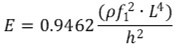(1)

where E is the modulus of elasticity of the specimen (Pa), is the air-dry density of the specimen (kgm-3), f1 is the first-order bending natural frequency of the specimen (Hz), L is the length of the specimen (m), and H is the thickness of the test piece (m).

By testing the first-order bending frequency f1 of the free beam, the elastic modulus value is calculated by Eq. 1.

Specimen and test methods

A total of 149 LVL specimens were used, with the dimensions of 2700 mm (l) 770 m (b)  30 mm (h). The air-dry density of specimens () was 480 to 618 kg/m3, and the moisture content (MC) was 9.5 to 14%.

Using the transient excitation vibration method, the LVL specification material specimen was struck with a rubber hammer to generate lateral free vibration. The frequency spectrum of the specimen was tested, and the first-order bending frequency of the specimen was identified from the spectrogram. The block diagram of LVL specification material specimen and test system is shown in Fig. 1.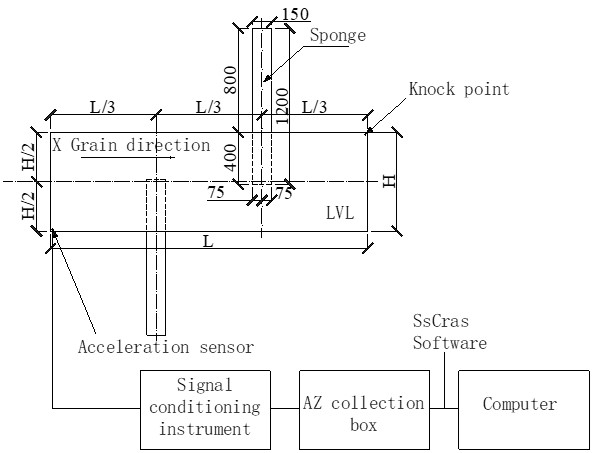Fig. 1. Block diagram of the fundamental frequency test system of the LVL test piece

Experimental protocol

The results of the modal test on the No. 2 specimen show that the ratio of the second, third, and fourth-order frequencies to the first-order frequency in the first four-order frequencies and the position of the first four-order mode nodes were completely consistent with their theoretical analytical solutions. Thus, the suspension tested in the article achieved free support. Therefore, a test plan for testing the first-order bending frequency of the specimen by the transient excitation method was proposed (Fu 2002; Wang et al. 2012), as follows:

1) Acquisition parameter settings: analysis frequency, 200 Hz; FFT length, 8192; acquisition mode, free running; one data block; voltage range, 5000 mV; and magnification of the conditioner, 100. The filter frequency was set to 200 Hz to prevent frequency aliasing, i.e., to ensure that the aliasing frequency (false frequency) of the third-order natural frequency did not appear on the spectrum.

2) The accelerometer was installed at the corner of the test specimen, and the rubber hammer hit the opposite corner of the test piece.

Figure 2 shows the frequency spectrum of LVL No. 2 specimen. It is not difficult to read the first-order bending frequency from the spectrogram; the fundamental frequency is 19.63 Hz.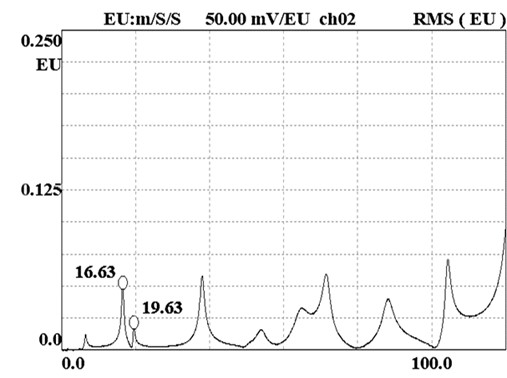Fig. 2. Free board sponge placement spectrum

The elastic modulus values of 149 LVL finished test pieces were measured by beam transverse bending vibration theory (Fig. 4). To illustrate the correctness of the test, the suspension method (Wang et al. 2015, 2018, 2019) was used for verification with a total of 20 samples. This method has proven to be accurate (Wang et al. 2015, 2018, 2019). Figure 3 shows that the elastic modulus measured by the two test methods has a good correlation.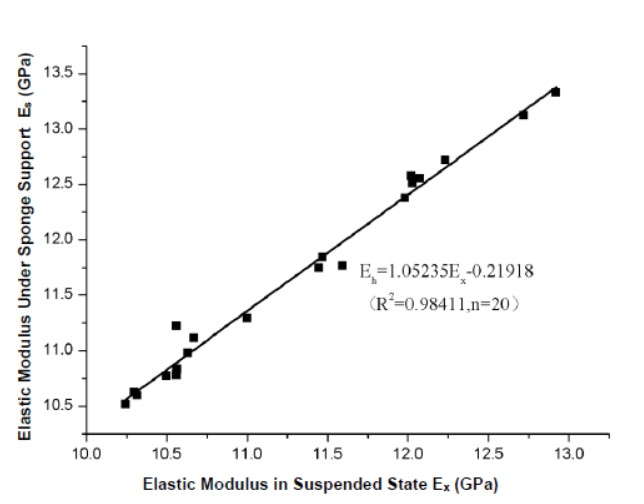Fig. 3. Correlation between elastic modulus in suspended state and elastic modulus under sponge support

Probability Distribution of LVL Elastic Modulus

Weibull distribution and its numerical characteristics (Weibull and Swenden 1951)

Given the elastic modulus E of LVL is a random variable , which conforms to the following three-parameter Weibull distribution function F(E),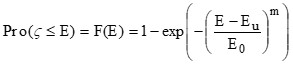,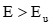(2)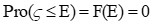where m and E0 are the distribution parameters, m is the shape parameter, E0 is the scale parameter, and Eu is the location parameter, which represents the overall minimum elastic modulus.

According to the definition of the overall average and standard deviation , and with the aid of the gamma function (x), Eqs. 3 and 4 are generated.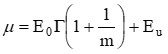(3)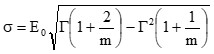(4)

Weibull distribution fit test

The measured values of the elastic modulus E of the sub-samples were arranged from small to large to form an order statistic Ei, i =1, 2, 3,……, 148, 149. The corresponding probability value is i/(n + 1). If the order statistic Ei has the same value and the number of repetitions is k, the corresponding probability value is (i * k)/(n + 1). The parameter n is the sample size, n =149. For a given Ei value, Eq. 2 shows that (Gumbel 1958):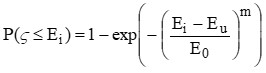(5)

Substituting the order statistic Ei and the corresponding probability value i/(n + 1) into Eq. 5, after logarithmic operation, produces Eq. 6.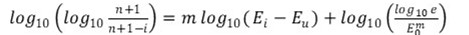(6)

According to the measured minimum elastic modulus E = 9.819 GPa, set Eu to be 0, 2.0, 2.5, 4.5, 6.0, 8.0, and 9.0G Pa, respectively. In the Weibull coordinate system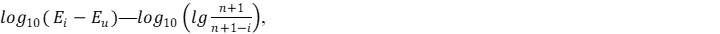the value of :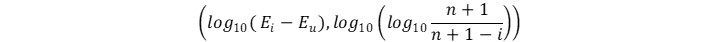is calculated. If the test point falls on a straight line, it is considered that the elastic modulus value can conform to the Weibull distribution, where m is determined by the slope of the straight line, and E0 is the intercept between the straight line and the coordinate axis.

The scatter plot of each measuring point in the Weibull coordinate system is shown in Fig. 4 (E = 9 GPa).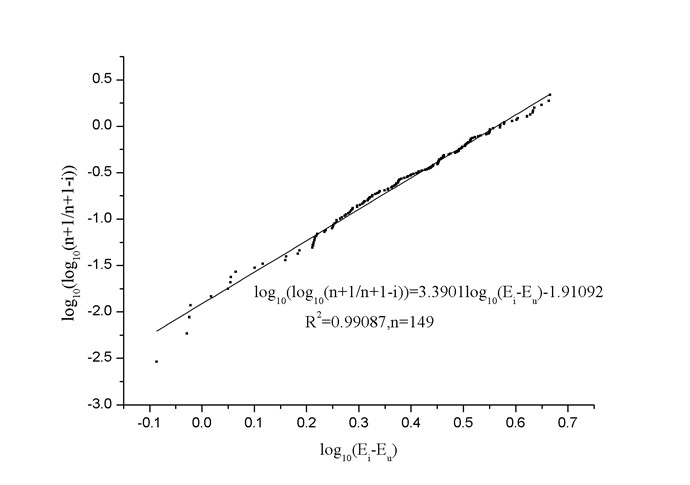Fig. 4. Fitting test of Weibull distribution of elastic modulus of LVL

When Eu = 9.0 GPa, R2 = 0.99087, n = 149, indicating that the fitting effect is good. Therefore, the elastic modulus of LVL in the Weibull coordinate system can be fitted with a straight line.

From the intercept of the straight line and the ordinate axis and the slope of the straight line, m = 3.3901, E0 = 2.86 GPa, the distribution function of LVL elastic modulus is as follows.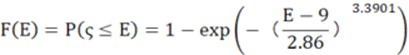Substituting m = 3.3901 and E0 = 2.86 GPa into Eqs. (2) and (3), the overall average m and standard deviation s are estimated to be: m=11.568 GPa; s=0.837 GPa; the coefficient of variation is 7.2%. For a sample with a capacity of 149, the sample average is 11.573 GPa, and the standard deviation is 0.848 GPa.

After the setting of the Eu value, the Weibull parameters obtained by linear fitting and the corresponding estimated LVL overall elastic modulus mean and standard deviation are shown in Table 1.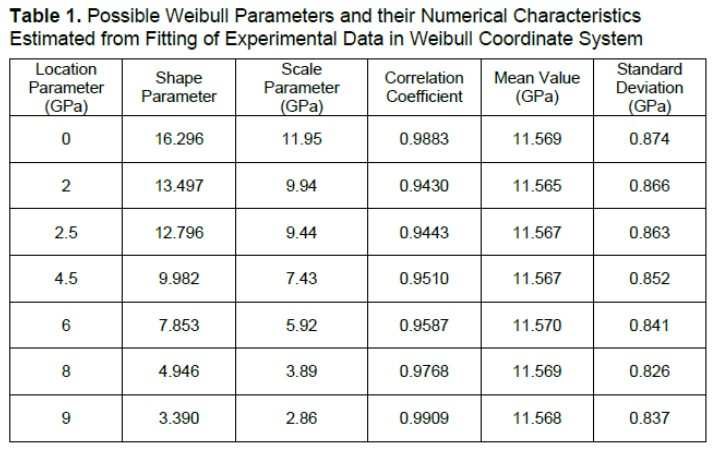K-S test of Weibull distribution and normal distribution

There are two types of random variable distribution type testing: known parameter estimation distribution type; unknown parameter, first estimate the parameter, and then test the distribution type. Among many inspection methods, the K-S method is the most commonly used one.

Test the hypothesis H0: LVL overall elastic modulus obeys Weibull distribution, H1: LVL elastic modulus does not obey Weibull distribution.

At present, the K-S test for testing hypothesis H0 belongs to the case of unknown parameters. First, the parameters should be estimated. The estimated values of the possible Weibull parameters are calculated from Table 1. One of the possible parameters corresponds to one of hypothetical known distribution function. Secondly, the difference between the hypothetical known distribution function and the empirical distribution function in the order statistics was calculated. The maximum value after taking the absolute value is the test statistic Dn value in the K-S method. Finally, according to the significance level α and the sample size, the critical value table of the Weibull distribution K-S test when the parameters are unknown was checked to obtain the critical value of the test statistic Dn,α, if Dn,α＞Dn, accept H0, otherwise reject H0 (Wang et al. 2015). The results of K-S test are shown in Table 2.

Using the sample mean and standard deviation as the estimated values of the population mean and standard deviation of the normal distribution, the K-S test was performed on the known distribution function of this hypothesis. The steps for calculating Dn are the same as above. The test results of the normal distribution are listed in Table 2.

The critical value d of Dn is obtained by looking up the table (Liang and Liu 1989; Wu et al. 1990; Ceraldi et al. 2001; Wu et al. 2001), based on the sample size and significance level α.

Weibull distribution:

=0.05, n=149,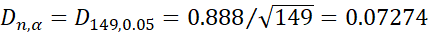(The parameters are unknown)

Normal distribution:

=0.05, n=149,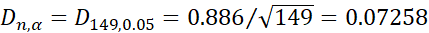(The parameters are unknown)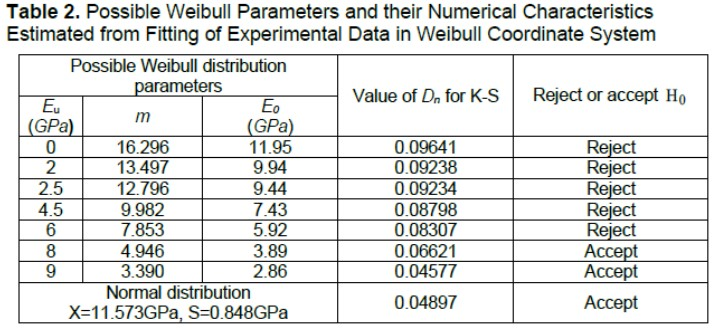The results in Table 2 show that: 1) the LVL elastic modulus does not obey the two-parameter Weibull distribution (Eu=0); 2) the LVL elastic modulus obeys the three-parameter Weibull distribution where the position parameter Eu is greater than half of the minimum test value; and 3) the LVL elastic modulus obeys the normal distribution.

Probability calculation of elastic modulus at a given value

The three-parameter Weibull distribution and normal distribution accepted by the K-S test are used to calculate the probability of elastic modulus at a given value (Li and Hu 2004). The results are shown in Table 3.

When 9 and 8 GPa were used as the setting values of Eu, the calculated probability value was relatively stable. At this time, Eu was 81% and 92%, respectively, of the minimum elastic modulus 9.815 GPa. Therefore, it was recommended to use 80% to 90% of the minimum value of the measured data as the setting value of the position parameter Eu. The three-parameter Weibull distribution and the normal distribution calculated LVL elastic modulus have the same probability under the given value.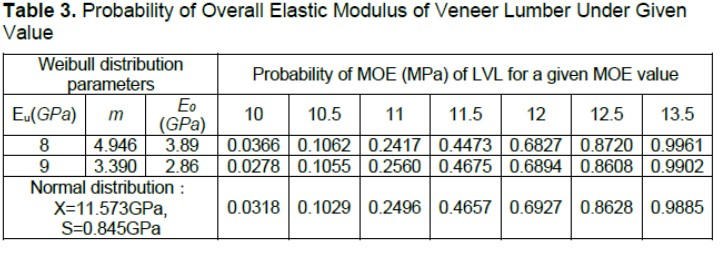To explain the probability characteristics of the calculation of the three-parameter Weibull distribution and the normal distribution, the probability distribution curve and probability density curve of the elastic modulus of LVL are given in Figs. 5 and 6.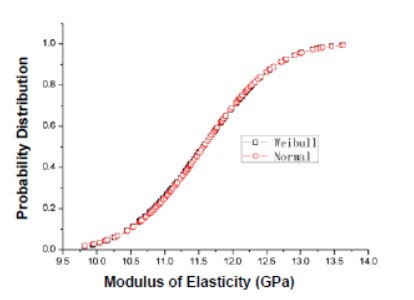Fig. 5. Probability distribution curve of elastic modulus of veneer laminated wood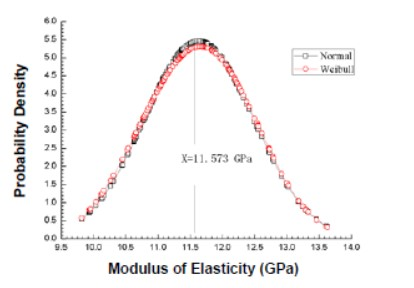Fig. 6. Probability density curve of elastic modulus of veneer laminated wood

Figures 5 and 6 show that 1) when the elastic modulus does not exceed 11 GPa, the normal probability distribution curve is slightly higher than the Weibull probability distribution curve, and when it exceeds 11 GPa, the opposite is true; 2) the Weibull probability density curve is slightly asymmetric with respect to its mean line (11.568 GPa), and the normal probability density curve is symmetric with respect to the mean line.

CONCLUSIONS

1. The modal test verifies that the test device in the article realizes the support condition of the free suspension of the test piece, and the test plan of the frequency method is proposed. The first-order bending and first-order torsional vibration natural frequency testing were used to calculate the elastic modulus of LVL.
2. The transient excitation frequency method to test the elastic modulus of LVL has the advantages of simplicity, speed, good repeatability, and high accuracy. Moreover, the frequency spectrum of LVL is basically the same in the state of free hanging and sponge support, and sponge can be used as the support condition of the test.
3. The elastic modulus of LVL obeys the normal distribution.
4. The probability that the elastic modulus of LVL calculated by the three-parameter Weibull distribution and the normal distribution is basically equal at a given value (accurate to the second decimal place).

ACKNOWLEDGEMENTS

This study was funded by The National Natural Science Foundation of China (51278106).

REFERENCES CITED

Boccadoro, L., Zweidler, S., Steiger, R., and Frangi, A. (2017). “Bending tests on timber-concrete composite members made of beech laminated veneer lumber with notched connection,” Engineering Structures 132, 14-28. DOI: 10.1016/j.engstruct.2016.11.029

Ceraldi, C., Mormone, V., and Ermolli, E. R. (2001). “Resistographic inspection of ancient timber structures for the evaluation of mechanical characteristics,” Materials & Structures 34, 59-64. DOI: 10.1007/BF02482201

Divino, E. T. (2016). “Evaluation of maximum strength and modulus of elasticity of Douglas fir lumber in axial to grain tension by two nondestructive techniques,” Revistaciência da Madeira 7(1), 1-6. DOI: 10.12953/2177-6830/rcm.v7n1p1-6

Fu, Z. F. (2002). Modal Analysis of Vibration and Parameter Identification, Chinese Machine Press, Beijing, China.

Gumbel, E. J. (1958). Statistics of Extremes, Columbia University Press, New York, USA.

Li, Y. Y., and Hu, C. R. (2004). Design and Data Processing, Chemical Industry Press, Beijing.

Liang, X. J., and Liu, G. H. (1989). “The fitting of the distribution of Kurtosis test statistics under the normal assumption,” Journal of East China Normal Univerity (Natural Science Edition) 01, 66-70.

Lv, Z. M., and Xu, J. W. (2001). “Identification method of dynamic natural frequency and damping coefficient of large-scale components,” Journal of Mechanical Engineering (06), 72-75+81. DOI: 10.3321/j.issn:0577-6686.2001.06.016

Nasir, V., Nourian, S., Avramidis, S., and Cool, J. (2019). “Stress wave evaluation by accelerometer and acoustic emission sensor for thermally modified wood classification using three types of neural networks,” European Journal of Wood and Wood Products 77, 45-55. DOI: 10.1007/s00107-018-1373-1

Purba, C. Y. C., Pot, G., Viguier, J., Ruelle, J., and Denaud, L. (2019). “The influence of veneer thickness and knot proportion on the mechanical properties of laminated veneer lumber (lvl) made from secondary quality hardwood,” European Journal of Wood and Wood Products 77, 393-204. DOI: 10.1007/s00107-019-01400-3

Schuler, T. A. (2017). “Toranoko Nursery laminated veneer lumber roof,” Architect 106(12), 53-56.

Wang, B. J., and Dai, C. P. (2013). “Development of structural laminated veneer lumber from stress graded crossmuk short-rotation hem-fir veneer,” Construction and Building Materials 47, 902-909. DOI: 10.1016/j.conbuildmat.2013.05.096

Wang, Z., Gu, L., Gao, Z., and Liu, B. (2015). “Dynamic testing and probability distribution of elastic modulus of SPF dimension lumbers,” Scientia Silvae Sinicae 51(2), 105-111.

Wang, Z., Li, L., and Gong, M. (2012). “Measurement of dynamic modulus of elasticity and damping ratio of wood-based composites using the cantilever beam vibration technique,” Construction & Building Materials 28(1), 831-834. DOI: 10.1016/j.conbuildmat.2011.09.001

Wang, Z., Xie, W. B., Lu, Y., Li, H. T., Wang, Z. H., and Li, Z. “Dynamic and static testing methods for shear modulus of oriented strand board,” Construction and Building Materials 216, 542-551. DOI: 10.1016/j.conbuildmat.2019.05.004

Wang, Z., Xie, W. B., Wang, Z. H., and Cao, Y. (2018). “Strain method for synchronous dynamic measurement of elastic, shear modulus and Poisson’s ratio of wood and wood composites,” Construction and Building Materials 182, 608-619. DOI: 10.1016/j.conbuildmat.2018.06.139

Wargua, U., Wojtkowiak, D., Kukla, M., and Talaka, K. (2020). “Symmetric nature of stress distribution in the elastic-plastic range of Pinus l. Pine wood samples determined experimentally and using the finite element method (FEM),” Symmetry, 13(1), 39. DOI: 10.3390/sym13010039

Weibull, W., and Swenden, S. (1951). “A statistical distribution of function wide applicability,” Journal of Applied Mechanics 18, 293-297.

Wu, S. W., and Ye, J. (1990). “Analysis of critical value of K-S test with unknown parameters,” Port Engineering Technology 01, 16-20.

Xie, W., Lu, Y., Wang, Z., Wang, X., Wu, X., and Gao, Z. (2019). “Feasibility of predictive assessment of bending performance of CLT plates of Canadian hemlock,” BioResources 14(3), 6047-6059. DOI: 10.15376/biores.14.3.6047-6059

Xue, B. (2013). Research on Mechanical Properties and Non-destructive Testing Reliability of Laminated Veneer Lumber, Master’s Thesis, Northeast Forestry University, Harbin, China.

Article submitted: Feb. 16, 2021; Peer review completed: Feb. 28, 2021; Revised version received and accepted: March 9, 2021; Published: March 19, 2021.

DOI: 10.15376/biores.16.2.3318-3327